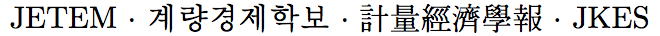Journal of Economic Theory and Econometrics: Journal of the Korean Econometric Society
Home

Archive

Search

Journal of Economic Theory and EconometricsJournal of the Korean Econometric Society

#### Natural Interest Rate, Potential GDP Growth Rate and Long-Term Monetary Policy StanceVol.31, No.2, June 2020, 40–69

English Version |  Korean Version
• #### Seonghoon Cho   (Yonsei University)Abstract

The natural rate of interest is the real interest rate that would prevail when the macro economy is in its natural, long-term equilibrium at which the actual GDP growth rate equals its potential rate. The natural rate of interest should therefore comove with the long-term trend of the potential GDP growth. In addition, since monetary policy authority has a significant impact on real interest rates, the difference between real and natural interest rates can be the basis for determining the long-term policy stance for the real sector of the economy. This study estimates potential growth rates and natural interest rates in Korea and the United States based on the Holston et al. (2017) model with important adjustments. We use the real market interest rate faced by economic agents, which may be different from the traditional real rate computed using the policy rate. Also, GDP per capita is considered, which is theoretically more suitable when population growth is taken into account. The estimation results using these new measures and the associated adjustment in the model reveal that the estimated natural rate of interest captures the theoretical characteristics of the natural interest rate better than the estimate using standard real interest rate. In addition, since the 2000s, Korea's real rate was much lower than the estimated natural interest rate, suggesting that the long-term monetary policy stance in Korea might have been strongly accommodating.

Keywords
Natural Rate of Interest, Potential Output Growth, Real Interest Rate, Long-term Monetary Policy Stance

JEL classification codes
C32, E43, E52